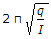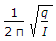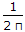# Mechanical Engineering - Theory of machines

### Exercise :: Theory of machines - Section 6

1.

The natural frequency of free torsional vibrations of a shaft is equal to (where q = Torsional stiffness of the shaft, and I = Mass moment of inertia of the disc attached at the end of a shaft)

 A.B. 2π qI C.D.Explanation:

No answer description available for this question. Let us discuss.

2.

If the rotating mass of a rim type flywheel is distributed on another rim type flywheel whose mean radius is half the mean radius of the former, then energy stored in the latter at the same speed will be

 A. four times the first one B. same as the first one C. one fourth of the first one D. one and a half times the first one

Explanation:

No answer description available for this question. Let us discuss.

3.

The Grubler's criterion for determining the degrees of freedom (n) of a mechanism having plane motion is (where l = Number of links, and j = Number of binary joints)

 A. n = (l - 1) - j B. n = 2(l - 1) - 2j C. n = 3(l - 1) - 2j D. n = 4(l - 1) - 3j

Explanation:

No answer description available for this question. Let us discuss.

4.

In a Hartnell governor, if a spring of greater stiffness is used, then the governor will be

 A. less sensitive B. more sensitive C. unaffected of sensitivity D. isochronous

Explanation:

No answer description available for this question. Let us discuss.

5.

The included angle for the V-belt is usually

 A. 10° to 20° B. 20° to 30° C. 30° to 40° D. 60° to 80°